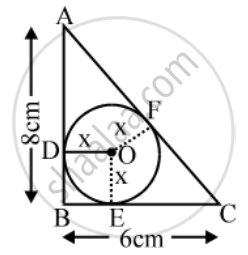# ABC is a right triangle, right angled at B. A circle is inscribed in it. The lengths of the two sides containing the right angle are 6 cm and 8 cm. Find the radius of the incircle. - Mathematics

Sum

ABC is a right triangle, right angled at B. A circle is inscribed in it. The lengths of the two sides containing the right angle are 6 cm and 8 cm. Find the radius of the incircle.

#### Solution

Let the radius of the in circle be x cm.Let the in circle touch the side AB, BC and CA at D, E, F respectively.

Let O be the centre of the circle.

Then, OD = OE = OF = x cm.

Also, AB = 8 cm and BC = 6 cm.

Since the tangents to a circle from an external point are equal, we have

AF = AD = (8 – x) cm, and

CF = CE = (6 – x) cm.

∴ AC = AF + CF = (8 – x) cm + (6 – x) cm

= (14 – 2x) cm.

Now ,

AC^2 = AB^2 + BC^2

⇒ (14 – 2x)^2 = 8^2 + 6^2 = 100 = (10)^2

⇒ 14 – 2x = ±10 ⇒ x = 2 or x = 12

⇒ x = 2 [neglecting x = 12].

Hence, the radius of the in circle is 2 cm.

Concept: Concept of Circle - Centre, Radius, Diameter, Arc, Sector, Chord, Segment, Semicircle, Circumference, Interior and Exterior, Concentric Circles
Is there an error in this question or solution?
Share